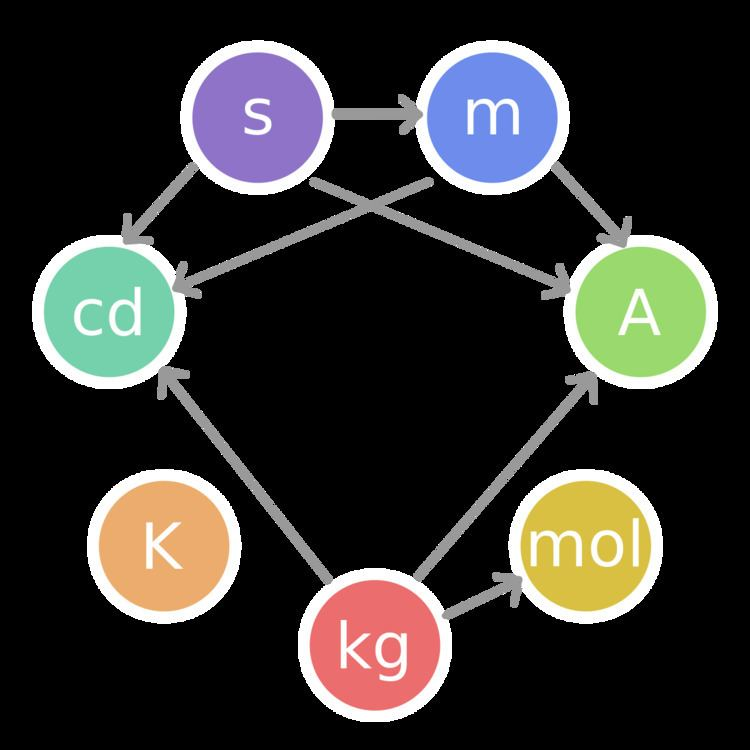# Length

Updated on
Edit
Like
CommentIn geometric measurements, length is the most extended dimension of an object. In the International System of Quantities, length is any quantity with dimension distance. In other contexts "length" is the measured dimension of an object. For example, it is possible to cut a length of a wire which is shorter than wire thickness.

## Contents

Length may be distinguished from height, which is vertical extent, and width or breadth, which are the distance from side to side, measuring across the object at right angles to the length. Length is a measure of one dimension, whereas area is a measure of two dimensions (length squared) and volume is a measure of three dimensions (length cubed). In most systems of measurement, the unit of length is a base unit, from which other units are defined.

## History

Measurement has been important ever since humans settled from nomadic lifestyles and started using building materials, occupying land and trading with neighbours. As society has become more technologically oriented, much higher accuracies of measurement are required in an increasingly diverse set of fields, from micro-electronics to interplanetary ranging.

One of the oldest units of length measurement used in the ancient world was the 'cubit' which was the length of the arm from the tip of the finger to the elbow. This could then be subdivided into shorter units like the foot, hand (which at 4 inches is still used today for expressing the height of horses) or finger, or added together to make longer units like the stride. The cubit could vary considerably due to the different sizes of people.

After Albert Einstein's special relativity, length can no longer be thought of being constant in all reference frames. Thus a ruler that is one meter long in one frame of reference will not be one meter long in a reference frame that is travelling at a velocity relative to the first frame. This means length of an object is variable depending on the observer.

## Units

In the physical sciences and engineering, when one speaks of "units of length", the word "length" is synonymous with "distance". There are several units that are used to measure length. Historically, units of length may have been derived from the lengths of human body parts, the distance travelled in a number of paces, the distance between landmarks or places on the Earth, or arbitrarily on the length of some fixed object.

In the International System of Units (SI), the basic unit of length is the metre and is now defined in terms of the speed of light. The centimetre and the kilometre, derived from the metre, are also commonly used units. In U.S. customary units, English or Imperial system of units, commonly used units of length are the inch, the foot, the yard, and the mile.

Units used to denote distances in the vastness of space, as in astronomy, are much longer than those typically used on Earth and include the astronomical unit, the light-year, and the parsec.

Length Wikipedia